Maths-
General
Easy

Question

# The Diameter of the moon is approximately one fourth of the diameter of the earth. What fraction of the volume of the earth is the volume of the moon?

## The correct answer is: 1/64

### Explanation: We have given the diameter of moon is approximately one fourth of the diameter of the earth. We have to find the fraction of the volume of the earth is the volume of the moon Step 1 of 1:Let the diameter of moon be ,Then the diameter of earth will be 4dSo, Radius of moon will be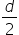. And the radius of earth will be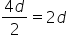Since, both earth and moon are sphereWe can use formula of volume of sphere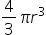.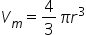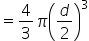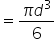And volume of earth will be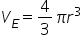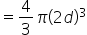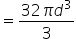Therefore, the fraction will be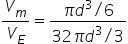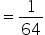#### With Turito Foundation.#### Get an Expert Advice From Turito.